Next: Feynman Rules for Tree Up: QED Processes Previous: Polarization in Electron Scattering

# Higher-Order Calculations: Tree and Loops

So far, we have considered the calculation of scattering amplitudes to lowest order in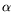. By putting together the vertices and propagators that we have found in these lowest-order calculations, we can find expressions for higher-order perturbation theory corrections and their corresponding Feynman diagrams. One such diagram is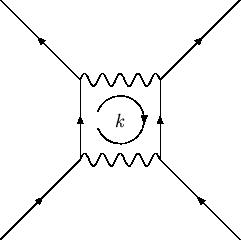By applying four-momentum conservation at each vertex one finds there is a free loop'' momentum,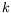. It is found that the correct procedure is to integrate over the loop momentum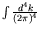. Unfortunately, most of the integrals that arise in the calculation of such loop diagrams are divergent and we have the paradoxical result that order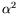corrections to the lowest-order scattering amplitude are infinite.

The precise programme for manipulating and taming'' these infinities is known as renormalization'' of the theory. Renomalization'' because all the infinities are miraculously swept up into formal expressions for the quantities like physical mass and charge of the particle.

We note however the useful distinction between tree'' diagrams - one with no loops - and loop'' diagrams - ones with one or more loops. For theories in which the physical coupling constant is small, such as, electromagnetic interactions, the tree diagram calculations are usually a good approximation to compare with experiment. Moreover, at the tree level, all the subtleties of renormailization never enter and so for many purposes it is sufficient to know how to calculate just these tree diagrams.Next: Feynman Rules for Tree Up: QED Processes Previous: Polarization in Electron Scattering
Douglas M. Gingrich (gingrich@ ualberta.ca)
2004-03-18